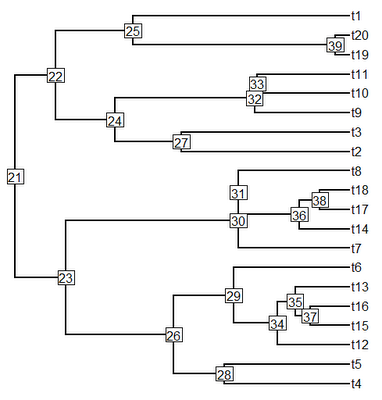## Thursday, February 23, 2012

### MRCA for a set of taxa

Do you know any function that could allow finding the deepest node ancestral to a group of tips? (I mean the oldest MRCA of a group of tips.)

The ape function mrca computes the pairwise most recent common ancestors for all the tips in a tree; however how do we take a set of pairwise MRCAs and identify the one that is also the MRCA of the whole group?

Well, by convention in "phylo" objects, node numbers increase along any path from the node. Logically, therefore, we ought to be able to pick the pairwise MRCA with the lowest number as the MRCA of a list. Since "phylo" objects are somewhat flexible, I decided to be careful and construct a function that pairs the ape function mrca with the phytools function nodeHeights to find the node in a set of pairwise MRCAs that is closest to the root node (i.e., it has the lowest height). Also, logically, this should be the MRCA of a set of tips.

The function looks as follows:

oldest.mrca<-function(tree,tips){
H<-nodeHeights(tree)
X<-mrca(tree)
n<-length(tips)
nodes<-height<-vector(); k<-1
for(i in 1:(n-1)) for(j in (i+1):n){
nodes[k]<-X[tips[i],tips[j]]
height[k]<-H[match(nodes[k],tree\$edge[,1]),1]
k<-k+1
}
z<-match(min(height),height)
return(nodes[z])
}

Let's load the function & phytools and then see how it works. First on an ultrametric tree (simulated using phytools pbtree):

> tree<-pbtree(n=20)
> plotTree(tree,node.numbers=T,pts=F)> oldest.mrca(tree,c("t14","t18")) # two taxa case
 36
> oldest.mrca(tree,c("t1","t3","t19"))
 22
> oldest.mrca(tree,c("t2","t3","t9","t11"))
 24
> oldest.mrca(tree,c("t2","t3","t4"))
 21

Now, let's try the same with a non-ultrametric tree:

> tree<-rtree(20)
> plotTree(tree,node.numbers=T,pts=F)> oldest.mrca(tree,c("t2","t12"))
 30
> oldest.mrca(tree,c("t14","t19","t20"))
 21
> oldest.mrca(tree,c("t10","t13","t20"))
 25

Seems to work. . . .

1.is it possible to find the mrca node without using nodeHeights?
I happen to have a clade (tr1 tips) from one tree and want to check that those species form the same clade in another tree( ie. find the ancestor and check that the descendants (tr2 tips) are the same in number and identity as tr1 tips.

2.Is there a reason to use NodeHeights from phytools instead of dist.nodes from ape? I substituted the latter (one less library since I am not using phytools for anything else), and it seems to work the same.

3.Is this now deprecated? I guess that the current implementation getMRCA(tree, tips) in 'ape' does exactly this? Or am I misunderstanding something?

1.Thanks for putting me onto this. oldest.mrca was never incorporated into phytools; however findMRCA was. I just updated (phytools 0.5-66 on GitHub) findMRCA to use getMRCA internally when it can. More info here.

Note: due to the very large amount of spam, all comments are now automatically submitted for moderation.